# Two marbles roll for eight seconds We observe

• Slides: 13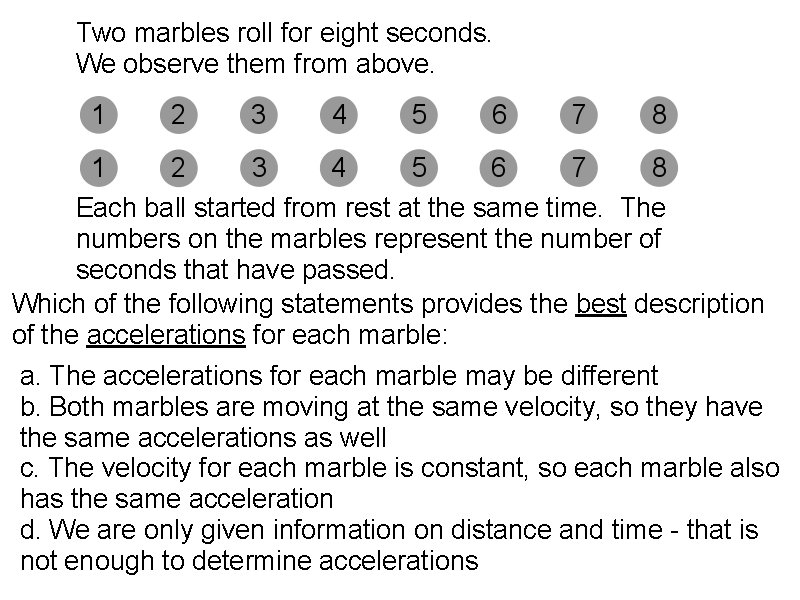Two marbles roll for eight seconds. We observe them from above. Each ball started from rest at the same time. The numbers on the marbles represent the number of seconds that have passed. Which of the following statements provides the best description of the accelerations for each marble: a. The accelerations for each marble may be different b. Both marbles are moving at the same velocity, so they have the same accelerations as well c. The velocity for each marble is constant, so each marble also has the same acceleration d. We are only given information on distance and time - that is not enough to determine accelerations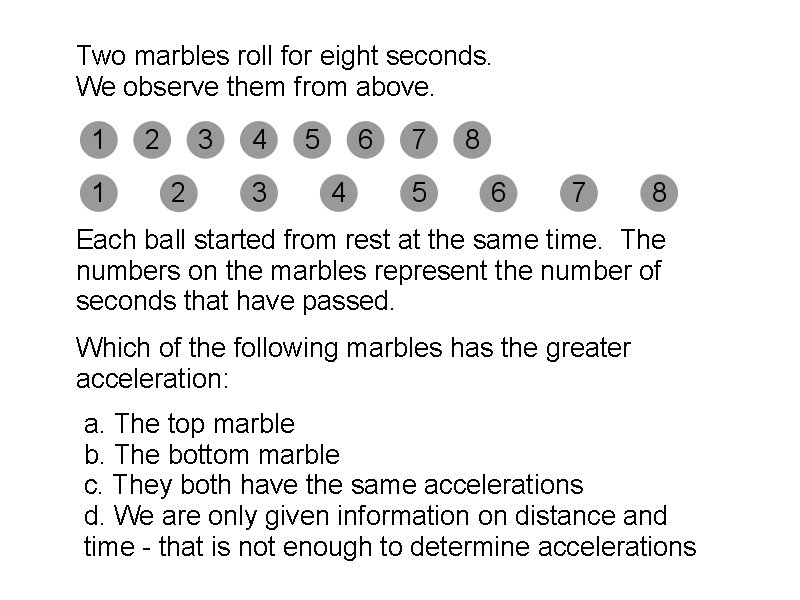Two marbles roll for eight seconds. We observe them from above. Each ball started from rest at the same time. The numbers on the marbles represent the number of seconds that have passed. Which of the following marbles has the greater acceleration: a. The top marble b. The bottom marble c. They both have the same accelerations d. We are only given information on distance and time - that is not enough to determine accelerations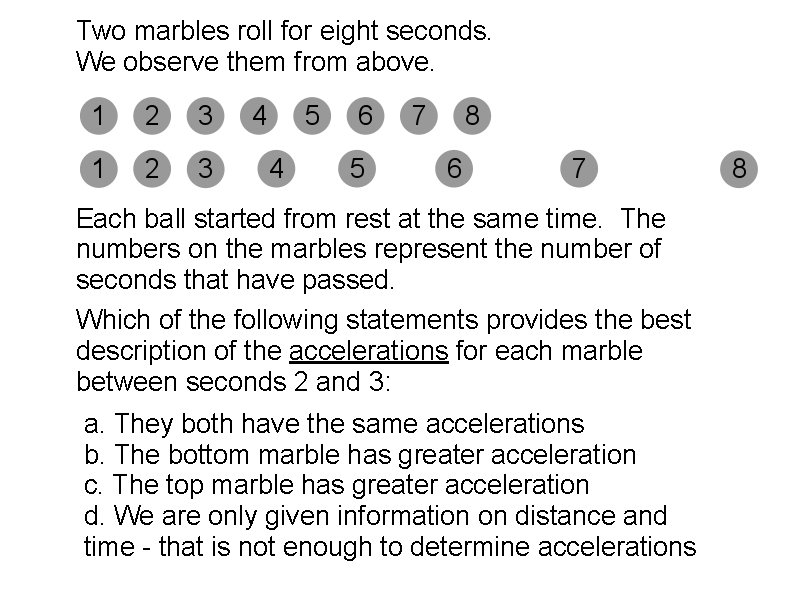Two marbles roll for eight seconds. We observe them from above. Each ball started from rest at the same time. The numbers on the marbles represent the number of seconds that have passed. Which of the following statements provides the best description of the accelerations for each marble between seconds 2 and 3: a. They both have the same accelerations b. The bottom marble has greater acceleration c. The top marble has greater acceleration d. We are only given information on distance and time - that is not enough to determine accelerations1 1 2 2 3 3 4 4 Both the tortoise and the hare started from rest at the same time. The numbers represent the number of seconds that have passed. Between 3 and 4 seconds, which of the following is true? a) The hare has greater average velocity and greater average acceleration b) The tortoise has greater average velocity and greater average acceleration c) The hare has greater average velocity and a lesser average acceleration d) The tortoise has lesser average velocity and a lesser average acceleration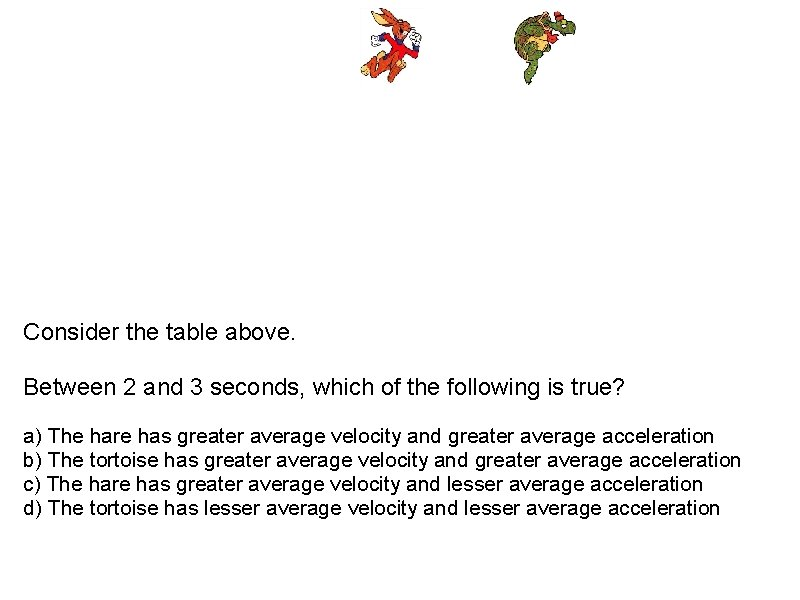Consider the table above. Between 2 and 3 seconds, which of the following is true? a) The hare has greater average velocity and greater average acceleration b) The tortoise has greater average velocity and greater average acceleration c) The hare has greater average velocity and lesser average acceleration d) The tortoise has lesser average velocity and lesser average acceleration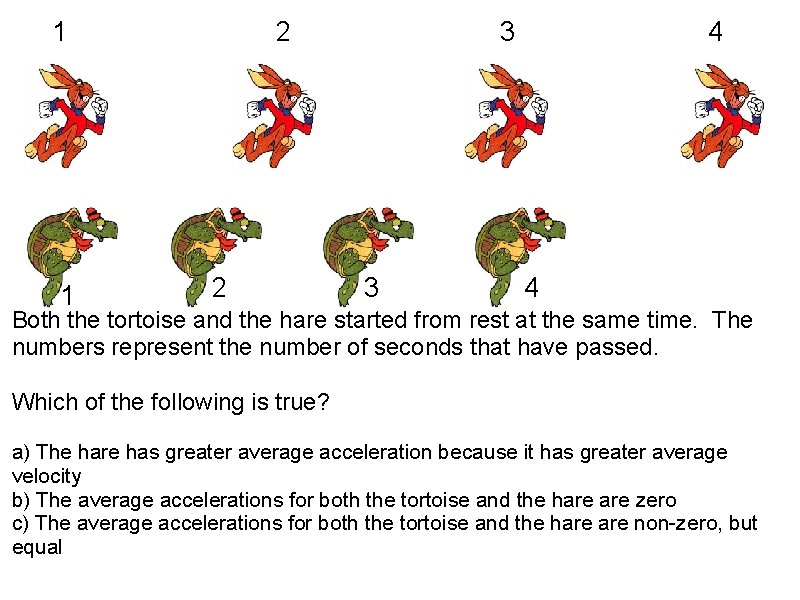1 1 2 2 3 3 4 4 Both the tortoise and the hare started from rest at the same time. The numbers represent the number of seconds that have passed. Which of the following is true? a) The hare has greater average acceleration because it has greater average velocity b) The average accelerations for both the tortoise and the hare zero c) The average accelerations for both the tortoise and the hare non-zero, but equal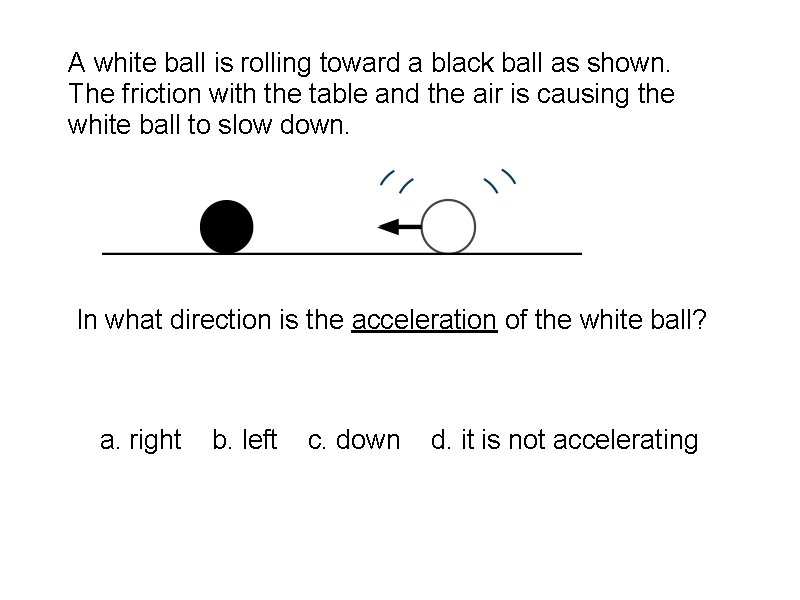A white ball is rolling toward a black ball as shown. The friction with the table and the air is causing the white ball to slow down. In what direction is the acceleration of the white ball? a. right b. left c. down d. it is not accelerating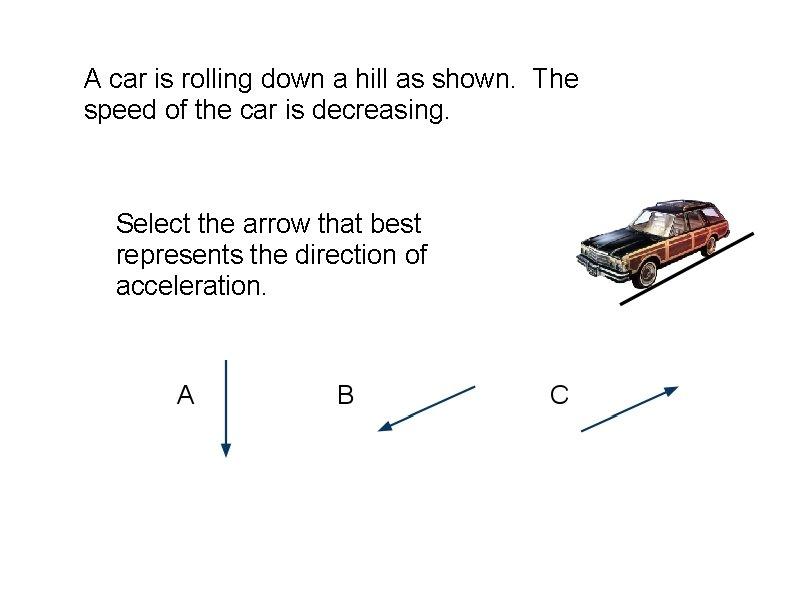A car is rolling down a hill as shown. The speed of the car is decreasing. Select the arrow that best represents the direction of acceleration.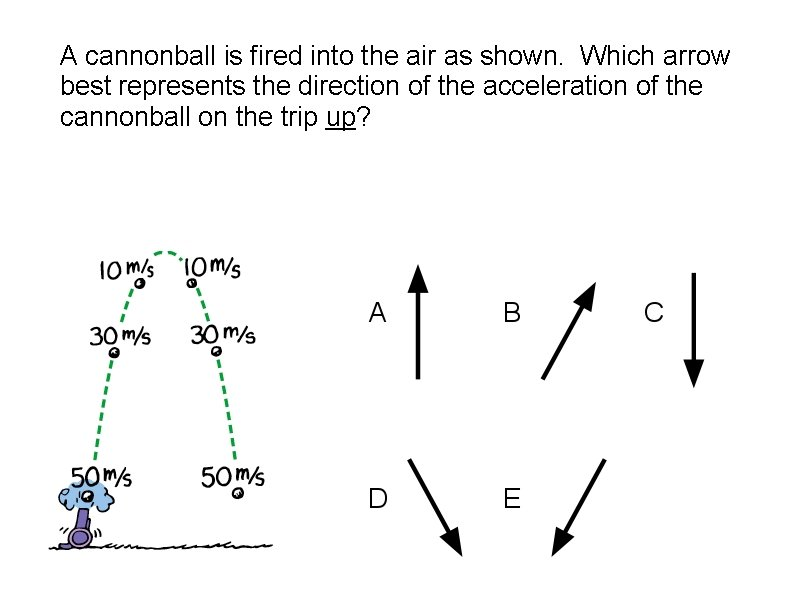A cannonball is fired into the air as shown. Which arrow best represents the direction of the acceleration of the cannonball on the trip up?A man is playing handball. When the ball strikes the wall it momentarily reaches zero velocity before bouncing back into the court. If we ignore gravity, the acceleration of the ball at this very moment is. . a. zero b. to the right c. to the left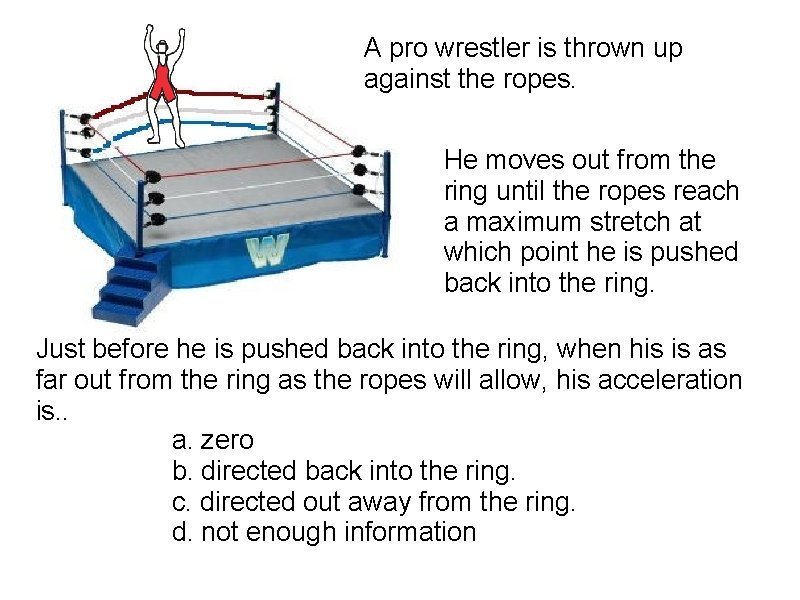A pro wrestler is thrown up against the ropes. He moves out from the ring until the ropes reach a maximum stretch at which point he is pushed back into the ring. Just before he is pushed back into the ring, when his is as far out from the ring as the ropes will allow, his acceleration is. . a. zero b. directed back into the ring. c. directed out away from the ring. d. not enough information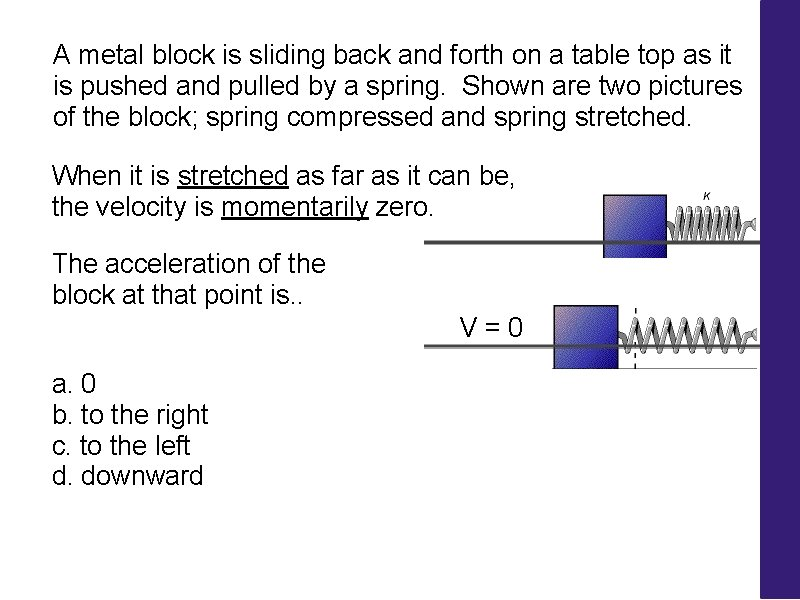A metal block is sliding back and forth on a table top as it is pushed and pulled by a spring. Shown are two pictures of the block; spring compressed and spring stretched. When it is stretched as far as it can be, the velocity is momentarily zero. The acceleration of the block at that point is. . V = 0 a. 0 b. to the right c. to the left d. downwardA bungee jumper is at the end of his cord. Just as the cord stretches to its maximum length, the jumper momentarily stops before bouncing back upward. The acceleration of the jumper at the moment in time described above is. . a. zero b. downward c. upward d. not enough information